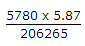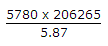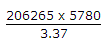Civil Engineering - UPSC Civil Service Exam Questions - Discussion

4.

In a trigonometric levelling, the height of the signal used is 4.62 m and the instrument height is 1.25 m. If the horizontal distance of the target from the instrument is 5780 m, then the axis-signal correction is

 [A].seconds [B].seconds [C].seconds [D].seconds

Explanation:

No answer description available for this question.

 Vai said: (Nov 3, 2017) Axis angle correction = (s2 - h1) / (D sin 1") = (s2 - h1) * 206265/ D. A is the correct answer.